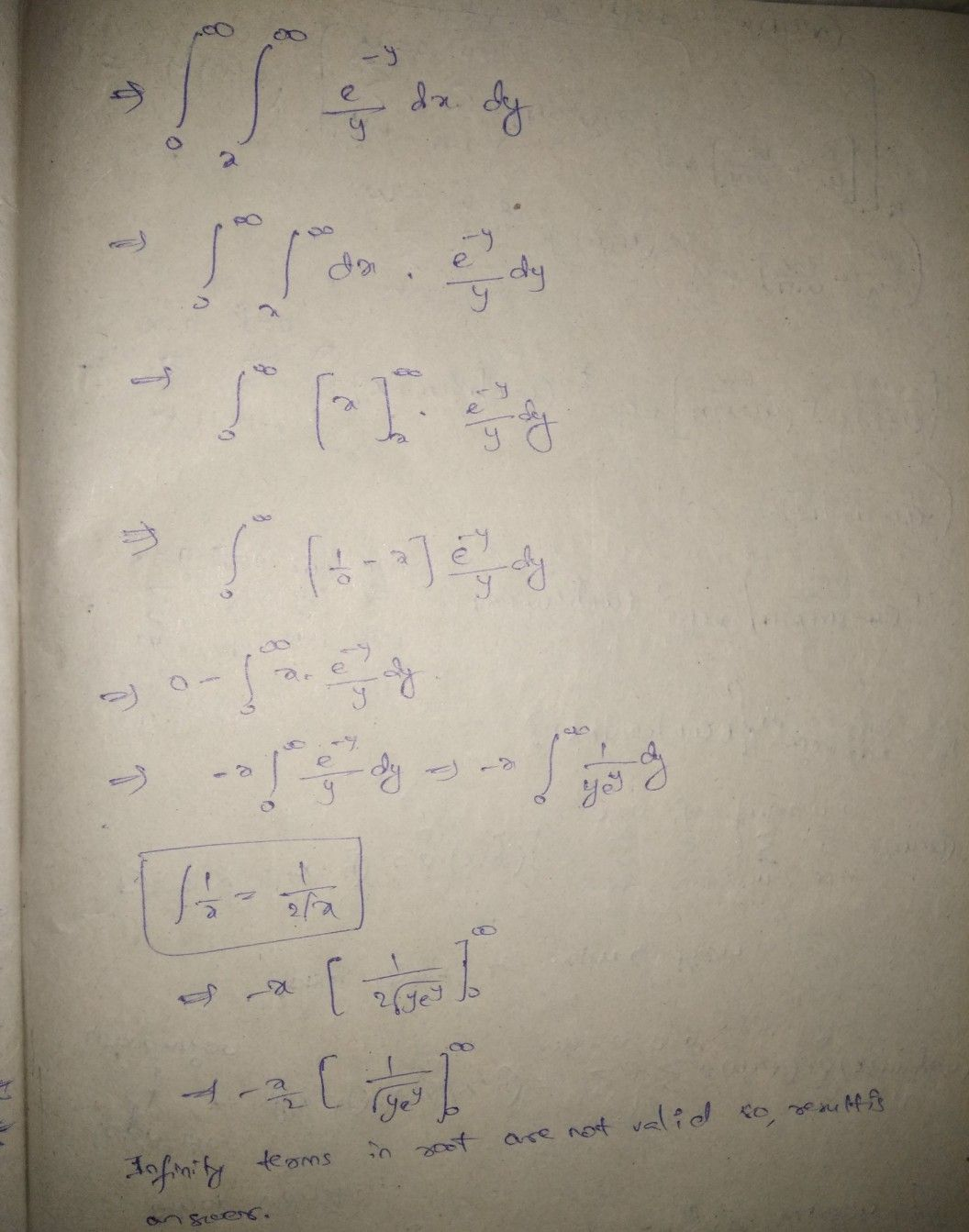Symbol
ProblemEvaluate the following by changing the eade $e$ of integsetion. $Q$ $\right)$ $-y$ $e$ $\dfrac {f} {0}f$ $x$ $y$ $dxd_{y}$
Calculus
SolutionQanda teacher - alekyago through itStudent
hello mam by changing orderQanda teacher - alekya
what